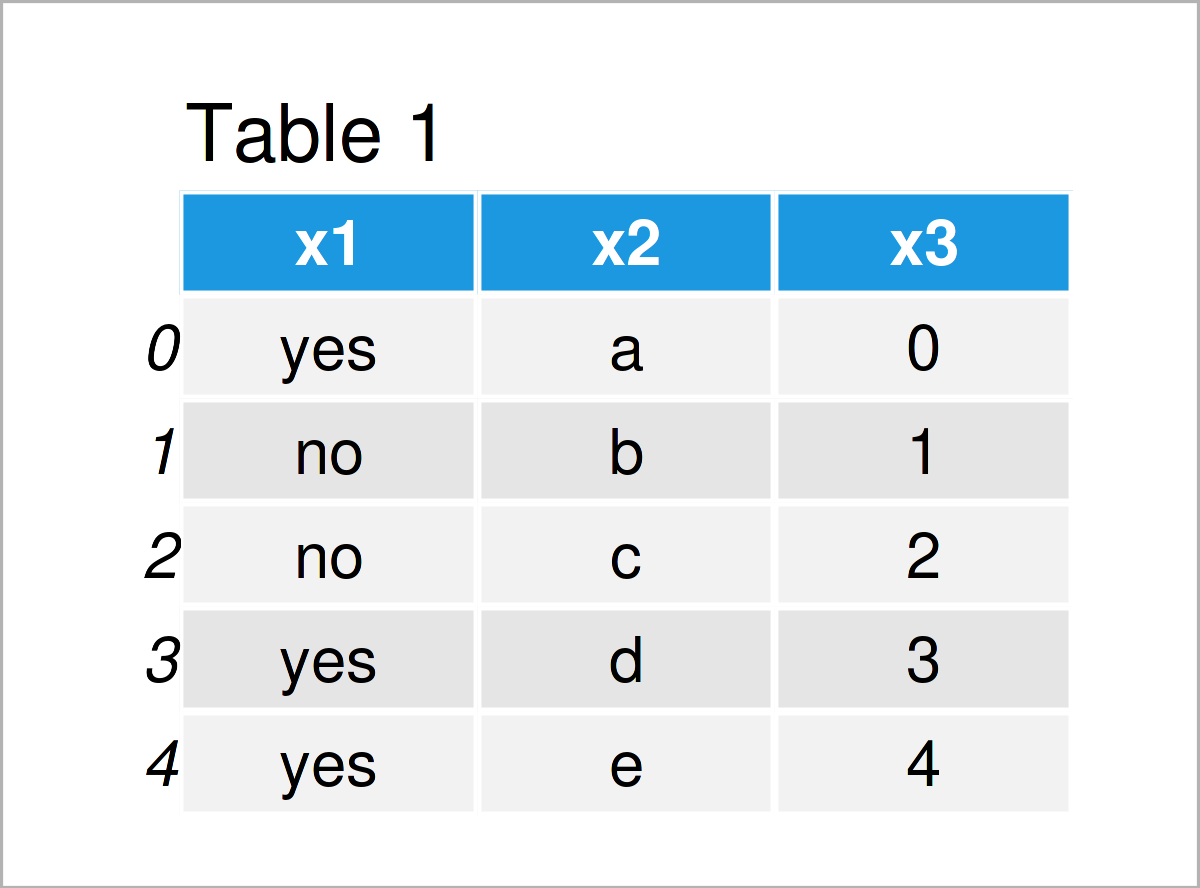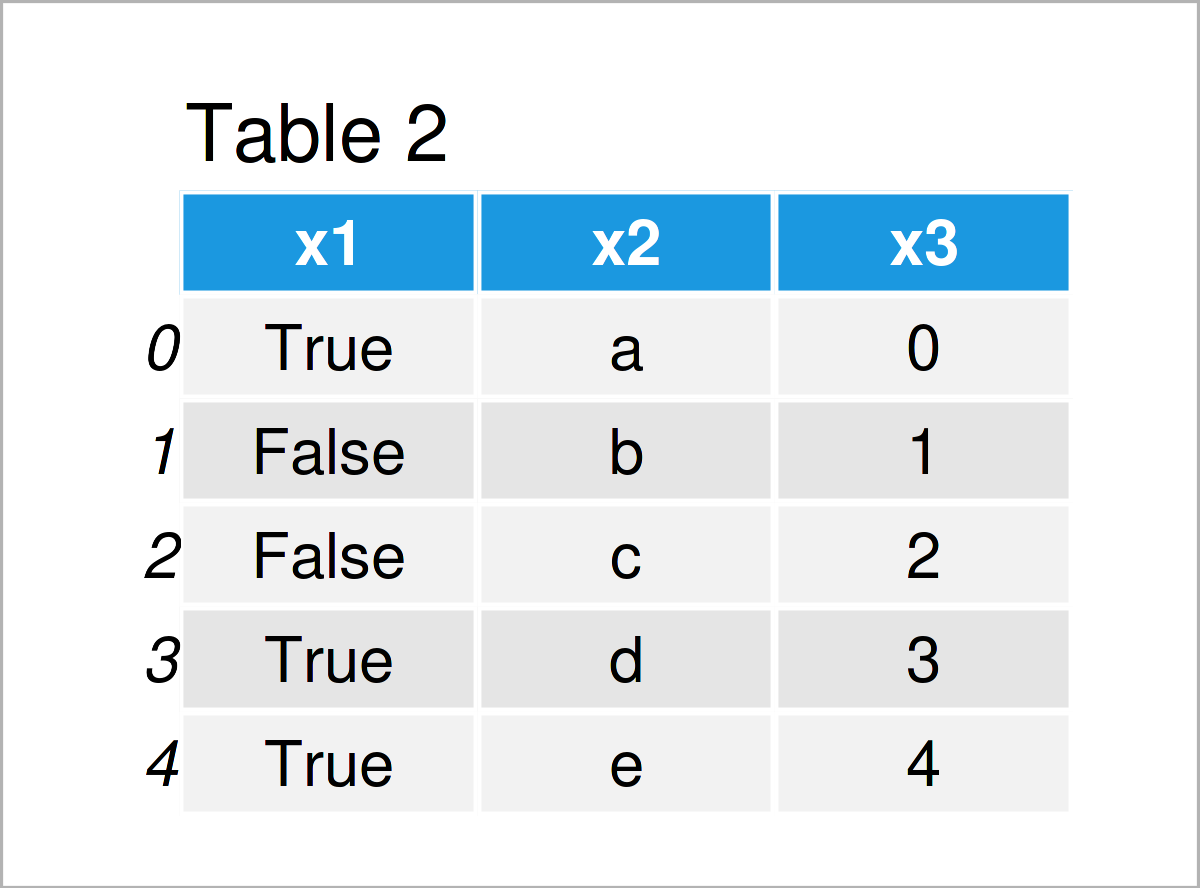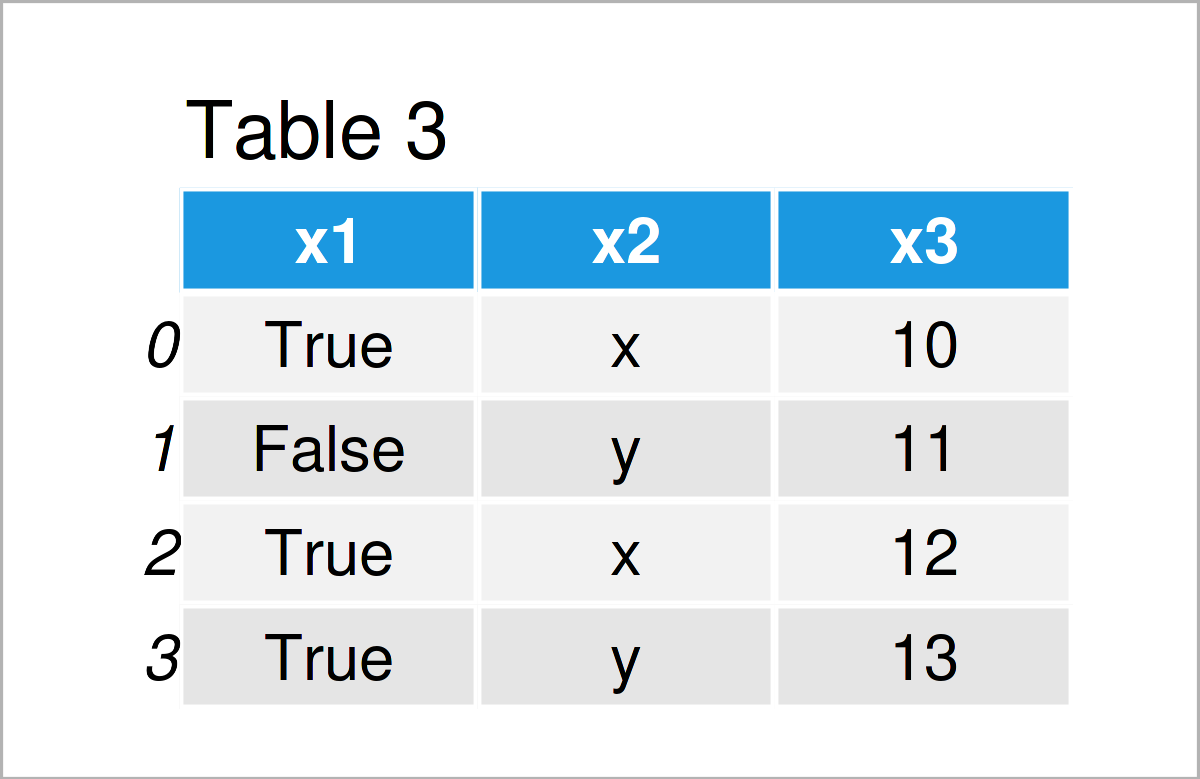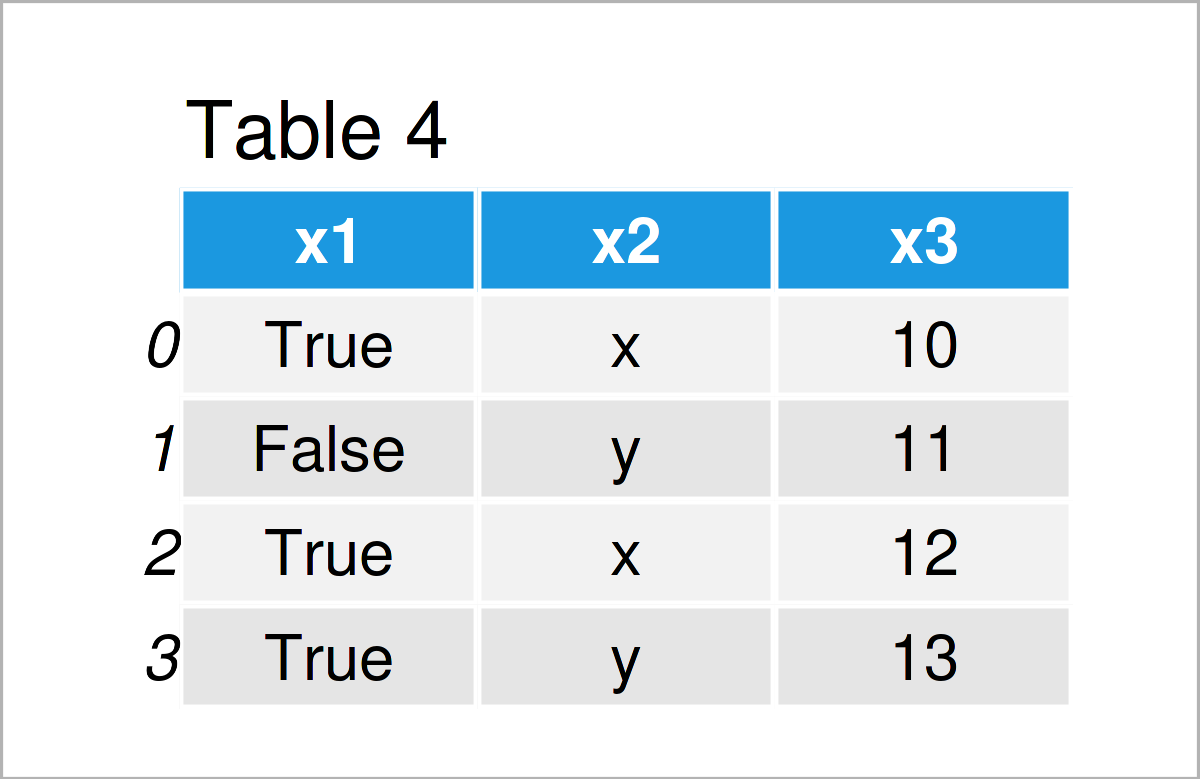# Convert String to Boolean in pandas DataFrame Column in Python (2 Examples)

In this article, I’ll demonstrate how to transform a string column to a boolean data type in a pandas DataFrame in Python programming.

Let’s dive right into the examples…

## Example 1: Replace String by Boolean in Column of pandas DataFrame

This section explains how to replace a string by a boolean data type in the column of a pandas DataFrame.

We first have to load the pandas library:

`import pandas as pd                                           # Load pandas library`

Next, we have to create a pandas DataFrame:

```data1 = pd.DataFrame({'x1':['yes', 'no', 'no', 'yes', 'yes'], # Create pandas DataFrame
'x2':['a', 'b', 'c', 'd', 'e'],
'x3':range(0, 5)})
print(data1)                                                  # Print pandas DataFrame```As shown in Table 1, we have constructed an exemplifying pandas DataFrame that contains three columns. The variables x1 and x2 are strings and the variable x3 is an integer.

Let’s assume that we want to convert the elements in the column x1 to the boolean class, i.e. the character string ‘yes’ should be converted to True and the character string ‘no’ should be changed to False.

Then, we can apply the map function as shown in Python code below:

```data1_bool = data1.copy()                                     # Create copy of pandas DataFrame
data1_bool['x1'] = data1_bool['x1'].map({'yes': True, 'no': False})      # Replace string by boolean
print(data1_bool)                                             # Print updated pandas DataFrame```After running the previous Python syntax the pandas DataFrame shown in Table 2 has been created. As you can see, the elements in the column x1 have been exchanged.

Let#s check the data types of the columns in our pandas DataFrame:

```print(data1_bool.dtypes)                                      # Check data types of columns
# x1      bool
# x2    object
# x3     int64
# dtype: object```

The previous output shows the classes of each column in our data set. As you can see, the first column x1 has the boolean data type.

## Example 2: Convert String Data Type to Boolean in Column of pandas DataFrame

In Example 2, I’ll demonstrate how to modify the class of a string column containing boolean expressions.

For this, we first have to create another pandas DataFrame:

```data2 = pd.DataFrame({'x1':['True', 'False', 'True', 'True'], # Create pandas DataFrame
'x2':['x', 'y', 'x', 'y'],
'x3':range(10, 14)})
print(data2)                                                  # Print pandas DataFrame```By running the previous Python programming code, we have created Table 3, i.e. another pandas DataFrame that contains boolean True and False values in the first column.

However, if we check the dtypes of our columns, we can see that the first column has the object class. Note that the pandas library stores strings in the object dtype.

```print(data2.dtypes)                                           # Check data types of columns
# x1    object
# x2    object
# x3     int64
# dtype: object```

If we want to change that, we can apply the map function once again:

```data2_bool = data2.copy()                                     # Create copy of pandas DataFrame
data2_bool['x1'] = data2_bool['x1'].map({'True': True, 'False': False})  # Replace string by boolean
print(data2_bool)                                             # Print updated pandas DataFrame```The output of the previous Python syntax is shown in Table 4: We have created a new pandas DataFrame that looks similar to the input DataFrame.

However, when we check the dtypes of the columns in this new data set, we can see the difference:

```print(data2_bool.dtypes)                                      # Check data types of columns
# x1      bool
# x2    object
# x3     int64
# dtype: object```

The variable x1 has been converted from string to boolean.

## Video & Further Resources

Do you need more info on the contents of this post? Then you could watch the following video tutorial on my YouTube channel. I’m explaining the topics of this article in the video:

In addition, you could have a look at some of the other Python articles that I have published on my homepage. I have released several articles already.

Summary: At this point you should have learned how to convert a character string column to a boolean data class in the Python programming language. In case you have additional questions, don’t hesitate to tell me about it in the comments. In addition, don’t forget to subscribe to my email newsletter for updates on the newest posts.

Subscribe to the Statistics Globe Newsletter